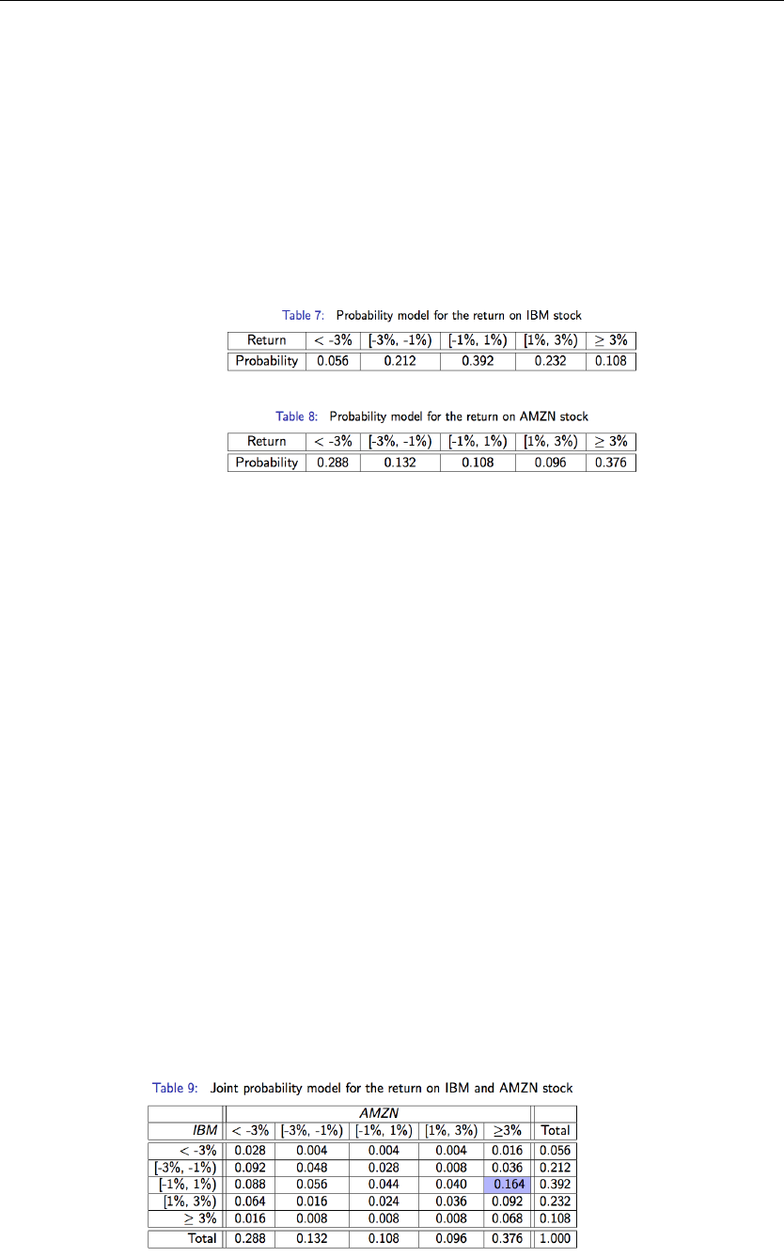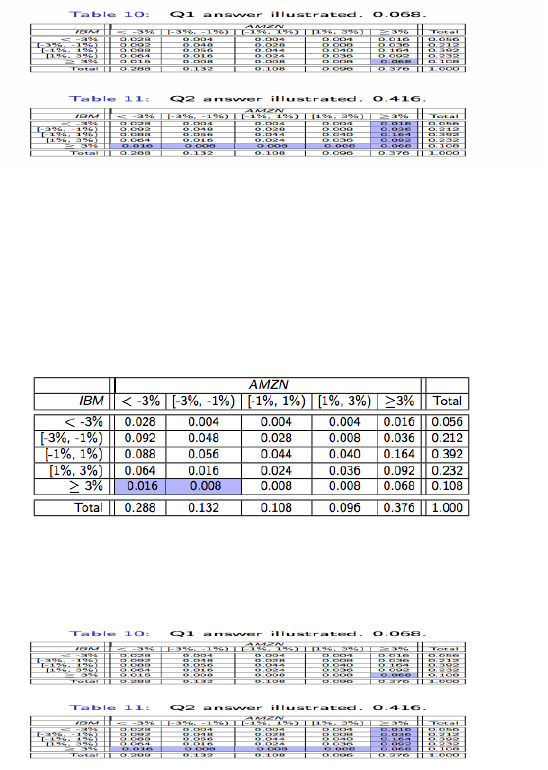Class Notes (1,100,000)
US (480,000)
UPenn (2,000)
STAT (70)
Lecture 8

# STAT 101 Lecture Notes - Lecture 8: Conditional Probability, Main Diagonal, Sample SpacePremium

Department
Statistics
Course Code
STAT 101
Professor
Richard Waterman
Lecture
8

This preview shows pages 1-2. to view the full 6 pages of the document.Stat 101 - Introduction to Business Statistics - Lecture 8: Continuing Probability
Marginal Probability
In some problems, the object of interest is the chance of two events happening
simultaneously. Another way of articulating this statement is that we are interested in
finding the association between two variables.
In the probability tables below we have probability models for the daily returns on two
stocks, IBM and Amazon.
If we make the assumption that the future looks like the past then we can use these
models to learn about future returns.
These distributions are called marginal probability distributions for each variable (not
telling you about how the two stocks move together)
The main thing to notice from these 2 tables is that AMZN is much more likely to have an
extreme return than is IBM. AMZN is described as more volatile, and this attribute can be
identified directly from the probability distribution of returns.
If one were to hold a portfolio of these 2 stocks then the behavior of the portfolio
depends on how the 2 stocks are related. This is a key concept used in optimal portfolio
construction in Finance and we will study its measurement shortly
Joint Probability
The probability table that describes the joint behavior of the two stock returns is called
the joint distribution.
The table on the next slide indicates for example that there is a probability of 0.164 that
AMZN goes up by over 3%, but IBM has a return of between -1% and 1%. (Note the
highlighted cell).
Notice that the row and column totals of the probability table are identical to the two
probability tables presented on Tables 7 and 8.
Probabilities inside the table are the probability of that combination of returns on stock
occurring (the probabilities on the edges are the marginal probabilities

Only pages 1-2 are available for preview. Some parts have been intentionally blurred.

Subscribers OnlyThere is much more chance that both stocks do well, or both do badly, rather
than one doing well and the other doing badly.
For example, the chances that both stocks go up by more than 3% is 0.068. The
chances that AMZN goes up by more than three percent, whereas IBM falls by
more than 3% is only 0.016.
It appears that the stocks move together to some extent. Again, more on this
shortly when we discuss covariance.
Lots of probability concentrated around leading diagonal indicating stocks are
positively correlated in their movements
We can now ask some questions about the performance of IBM and AMZN
simultaneously.
Q1 What is the probability that AMZN has a return of more than 3% AND IBM has
a return of more than 3%. → 0.068.
Q2 What is the probability that AMZN has a return of more than 3% OR IBM has
a return of more than 3%. → 0.416.
for this question, you can either add highlighted cells or add probability of
AMZN obtaining more than 3%, IBM obtaining more than 3% and then
subtracting the probability of both being more than 3% (union of both)
Q3 What is the probability that AMZN has a return of less than -1% AND IBM has
a return of NOT less than 3%. → 0.024.
All these questions can be answered by returning to the joint distribution table
and identifying the events that correspond to the questions. The probabilities of
these “simple” events are then summed to find the answer